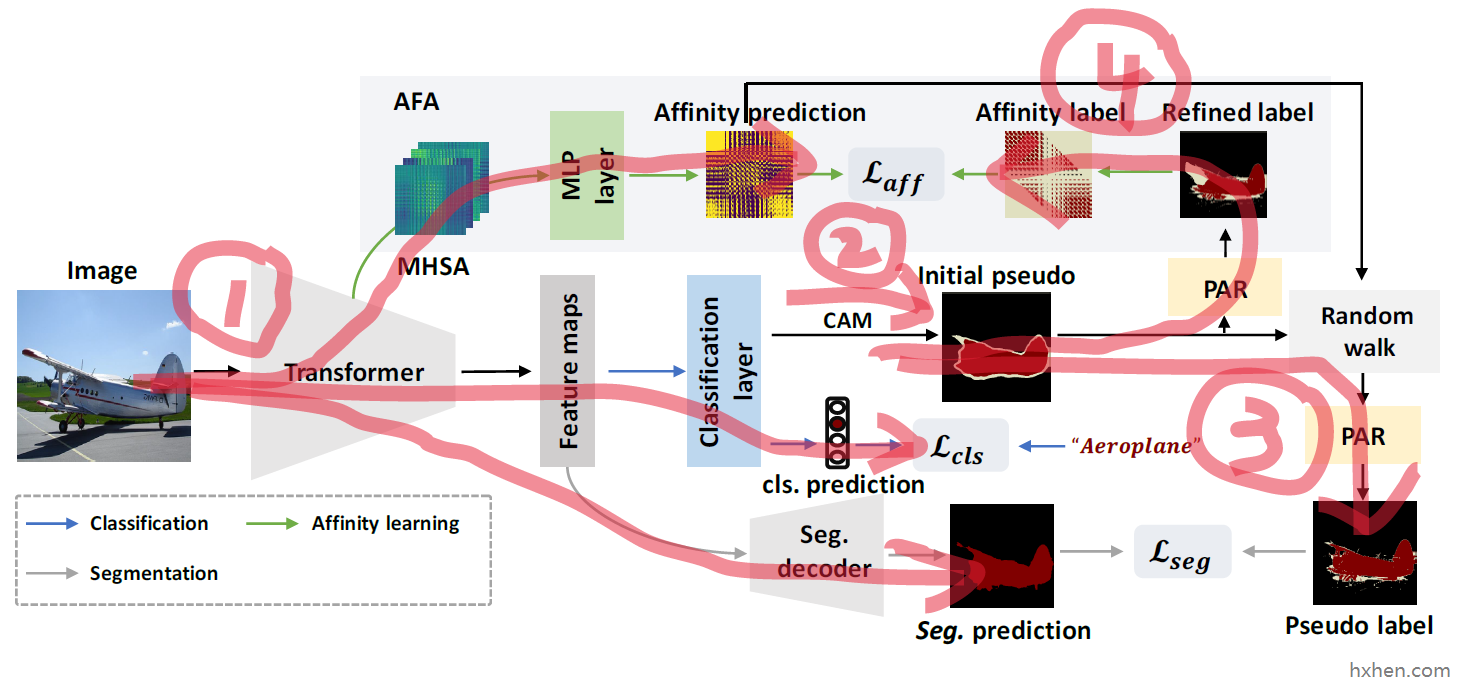# 简记：Learning Affinity from Attention: End-to-End Weakly-Supervised Semantic Segmentation with Transformers

Learning Affinity from Attention End-to-EndWeakly-Supervised Semantic

# 简记：Learning Affinity from Attention: End-to-End Weakly-Supervised Semantic Segmentation with Transformers

### 创新点描述

• 提出了端到端的、只利用图像级标签的、基于Transformer的 弱监督语义分割框架
• 提出了 Affinity from Attention (AFA) 模块，用于从Transformer的多头自注意力（MHSA）中学得语义亲和度（semantic affinity）的信息，用于 propagate （传播/扩散/生长）伪标签（pseudo label）
• 提出了 Pixel-Adaptive Refinement (PAR) 模块，用于 incorporate （合并/混合）图像的颜色信息（RGB）和空间信息（像素坐标），用于优化伪标签

### 整体结构• 路径一是Transformer输出三个数据：预测的类别 classification prediction，预测的分割结果 segmentation prediction，预测的亲和度矩阵 affinity prediction。其中类别预测结果是直接使用已有的图像级标签来进行监督的。分割结果和亲和度矩阵的监督信息需要通过后面三个部分获得。
• 路径二是生成类激活图（Class Activation Map，CAM），并基于CAM生成初始伪标签。
• 路径三是对初始伪标签进行优化，主要用到 random walker 算法和 PAR 模块，优化后得到最终伪标签，用于监督分割结果的生成。
• 路径四是先对初始伪标签进行优化，主要用到 PAR 模块，使用优化后的伪标签生成亲和度矩阵的标签，用于监督亲和度矩阵的生成。

### 路径一：Transformer编码器的三个输出结果

#### 亲和度矩阵的生成

$\begin{array}{}\text{(1)}& A=\mathbf{M}\mathbf{L}\mathbf{P}\left(S+{S}^{T}\right)\end{array}$

### 路径二：CAM的生成、初始伪标签的生成

#### CAM的生成

Transformer作为编码器，每幅输入的图像都会对应一组它输出的特征图 $F\in {\mathbb{R}}^{hw×d}$$F\in \mathbb{R}^{hw\times d}$ ，这里的特征是 $d$$d$ 维的，经过图中的 Classification layer 之后得到 $c$$c$ 维的CAM图 $M\in {\mathbb{R}}^{h×w×c}$$M\in \mathbb{R}^{h\times w\times c}$ 。（从程序上看图中的 Classification layer 也是单层卷积）

$\begin{array}{}\text{(2)}& {M}^{c}=\mathbf{R}\mathbf{e}\mathbf{L}\mathbf{u}\left(\sum _{i=1}^{d}{W}^{i,c}{F}^{i}\right)\end{array}$

#### 初始伪标签的生成

• 低于 ${\beta }_{l}$$\beta_l$ 的区域置为 0，表示可信背景区域
• 高于 ${\beta }_{h}$$\beta_h$ 的区域使用 argmax 获得各像素位置的类别。如：任务有4个类别，则这部分区域的取值范围为 $\left\{1,2,3,4\right\}$$\{1,2,3,4\}$
• 介于两者之间的区域置为 255，表示不确定区域，不予处理

$\begin{array}{}\text{(3)}& \begin{array}{c}{Y}_{p}^{i,j}=\left\{\begin{array}{rlr}& \mathrm{a}\mathrm{r}\mathrm{g}\mathrm{m}\mathrm{a}\mathrm{x}\left({M}^{i,j,:}\right),& \mathrm{i}\mathrm{f}max\left({M}^{i,j,:}\right)\ge {\beta }_{h},\\ & 0,& \mathrm{i}\mathrm{f}max\left({M}^{i,j,:}\right)\le {\beta }_{l},\\ & 255,& \mathrm{o}\mathrm{t}\mathrm{h}\mathrm{e}\mathrm{r}\mathrm{w}\mathrm{i}\mathrm{s}\mathrm{e},\end{array}\end{array}\end{array}$

### 路径三：最终伪标签的生成

#### 伪标签的扩散传播

$\begin{array}{}\text{(4)}& \begin{array}{rl}& {M}_{aff}=T\cdot \mathrm{v}\mathrm{e}\mathrm{c}\left(M\right)\\ \\ & T={D}^{-1}{A}^{\alpha }\\ \\ & {D}^{i,i}=\sum _{k}{A}^{{\alpha }^{i,k}}\end{array}\end{array}$

#### PAR模块对伪标签的优化### 路径四：亲和度矩阵标签的生成

• 如果 $i,j$$i, j$ 都是确定区域

• 如果 ${Y}_{p}^{i}$$Y_p^{i}$${Y}_{p}^{j}$$Y_p^{j}$ 取值相同，则 ${Y}_{aff}^{i,j}$$Y_{aff}^{i,j}$ 置为 1，同时位置 $i,j$$i,j$ 记为positive位置对；
• 如果 ${Y}_{p}^{i}$$Y_p^{i}$${Y}_{p}^{j}$$Y_p^{j}$ 取值不相同，则 ${Y}_{aff}^{i,j}$$Y_{aff}^{i,j}$ 置为 0，同时位置 $i,j$$i,j$ 记为negative位置对；
• 如果 $i$$i$$j$$j$ 任意一方为不确定区域，则 ${Y}_{aff}^{i,j}$$Y_{aff}^{i,j}$ 置为 255，表示“忽略”

• 此外，邻域外的关系均置为 255，且 ${Y}_{aff}^{i,j}$$Y_{aff}^{i,j}$${Y}_{aff}^{j,i}$$Y_{aff}^{j,i}$ 取值相同。

### 损失函数

$\begin{array}{}\text{(5)}& \mathcal{L}={\mathcal{L}}_{cls}+{\lambda }_{1}{\mathcal{L}}_{seg}+{\lambda }_{2}{\mathcal{L}}_{aff}+{\lambda }_{3}{\mathcal{L}}_{reg}\end{array}$

$\begin{array}{}\text{(6)}& \begin{array}{rl}{\mathcal{L}}_{aff}& =\frac{1}{{N}^{+}}\sum _{\left(ij,kl\right)\in {\mathcal{R}}^{+}}\left(1-\mathrm{s}\mathrm{i}\mathrm{g}\mathrm{m}\mathrm{o}\mathrm{i}\mathrm{d}\left({A}^{ij,kl}\right)\right)\\ & +\frac{1}{{N}^{-}}\sum _{\left(ij,kl\right)\in {\mathcal{R}}^{-}}\mathrm{s}\mathrm{i}\mathrm{g}\mathrm{m}\mathrm{o}\mathrm{i}\mathrm{d}\left({A}^{ij,kl}\right)\end{array}\end{array}$

（按照我的理解，文章中的公式应该是将加号代表的positive项和减号代表的negative项写反了，所以上面式子的加减号上标和原文刚好相反，如理解不当望指出。）